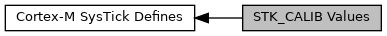libopencm3 A free/libre/open-source firmware library for various ARM Cortex-M3 microcontrollers.
STK_CALIB Values
Collaboration diagram for STK_CALIB Values:## Macros

#define STK_CALIB_NOREF   (1 << 31)
NOREF: NOREF flag Bit31 => NOREF Indicates whether the IMPLEMENTATION DEFINED reference clock is implemented: 0 = The reference clock is implemented. More...

#define STK_CALIB_SKEW   (1 << 30)
SKEW: SKEW flag Bit30 => SKEW Indicates whether the 10ms calibration value is exact: 0 = 10ms calibration value is exact. More...

#define STK_CALIB_TENMS   0x00FFFFFF
TENMS Calibration value for 10ms. More...

## ◆ STK_CALIB_NOREF

 #define STK_CALIB_NOREF   (1 << 31)

NOREF: NOREF flag Bit31 => NOREF Indicates whether the IMPLEMENTATION DEFINED reference clock is implemented: 0 = The reference clock is implemented.

1 = The reference clock is not implemented. When this bit is 1, the CLKSOURCE bit of the SYST_CSR register is forced to 1 and cannot be cleared to 0.

Definition at line 158 of file systick.h.

## ◆ STK_CALIB_SKEW

 #define STK_CALIB_SKEW   (1 << 30)

SKEW: SKEW flag Bit30 => SKEW Indicates whether the 10ms calibration value is exact: 0 = 10ms calibration value is exact.

1 = 10ms calibration value is inexact, because of the clock frequency

Definition at line 165 of file systick.h.

## ◆ STK_CALIB_TENMS

 #define STK_CALIB_TENMS   0x00FFFFFF

TENMS Calibration value for 10ms.

Bit0 to 23 => TENMS Optionally, holds a reload value to be used for 10ms (100Hz) timing, subject to system clock skew errors. If this field is zero, the calibration value is not known.

Definition at line 173 of file systick.h.